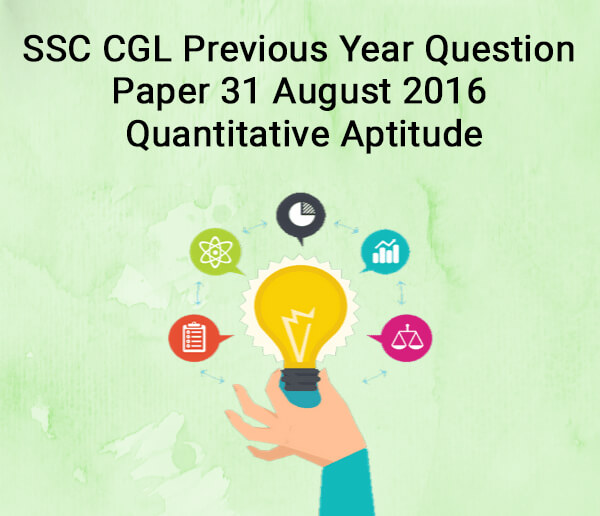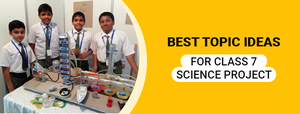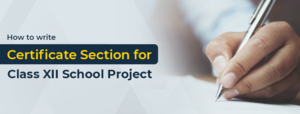SSC CGL Previous Year Question Paper 31 August 2016 - Quanti - Study24x7Default error msg

New to Study24x7 ?
By continuing, you agree to Study24x7 ?

# SSC CGL Previous Year Question Paper 31 August 2016 - Quantitative Aptitude

Updated on 15 February 2020SSC Preparation Strategies & S
Updated on 15 February 2020Question 1.A and B together can do a piece of work in 9 days. If A does thrice the work of B in a given time, the time A alone will take to finish the work is

a. 4 days

b. 6 days

c. 8 days

d. 12 days

Question 2.The diameters of two cylinders are in the ratio 3:2 and their volumes are equal. The ratio of their heights is

a. 2:3

b. 3:2

c. 9:4

d. 4:9

Question 3.A trader sold a cycle at a loss of 10%. If the selling price had been increased by Rs. 200, there would have been a gain of 6%. The cost price of the cycle is

a. Rs.1200

b. Rs.1205

c. Rs.1250

d. Rs.1275

Question 4.In a city, 40% of the people are illiterate and 60% are poor. Among the rich, 10% are illiterate. The percentage of the illiterate poor population is

a. 36

b. 60

c. 40

d. 50

Question 5.In what time will a 100 metres long train running with a speed of 50 km/hr cross a pillar?

a. 7.0 sec

b. 72 sec

c. 7.2 sec

d. 70 secQuestion 7.If l + m + n = 9 and l2 + m2 + n2 = 31, then the value of lm + mn + nl will be

a. 22

b. 50

c. 25

d. -25

Question 8.The centroid of a triangle is the point where

a. the medians meet

b. the altitudes meet

c. the right bisectors of the sides of the triangle meet

d. the bisectors of the angles of the triangle meet

Question 9.In a triangle PQR, the side QR is extended to S. ∠QPR = 72° and ∠PRS = 110°, then the value of ∠PQR is:

a. 38°

b. 32°

c. 25°

d. 29°

Question 10.In a trapezium ABCD, AB || CD, AB < CD, CD = 6 cm and distance between the parallel sides is 4 cm. If the area of ABCD is 16 cm2, then AB is

a. 1 cm

b. 2 cm

c. 3 cm

d. 8 cm

Question 11.If tanθ + cotθ = 5, then the value of tan2 θ+ cot2 θ is

a. 22

b. 25

c. 23

d. 27

Question 12.When a number is divided by 56, the remainder will be 29. If the same number is divided by 8, then the remainder will be

a. 6

b. 7

c. 5

d. 3

Question 13.If a shop keeper marks his goods for a certain amount so as to get 25% gain after allowing a discount of 20%, then his marked price is

a. Rs.156.25

b. Rs.146.25

c. Rs.166.67

d. Rs.150.25

Question 14.The average of marks of 17 students in an examination was calculated as 71. But it was later found that the mark of one student had been wrongly entered as 65 instead of 56 and another as 24 instead of 50. The correct average is

a. 70

b. 71

c. 72

d. 73

Question 15.The simple interest on a sum for 5 years is two-fifth of the sum. The rate of interest per annum is

a. 0.1

b. 0.08

c. 0.06

d. 0.04Question 17.If a - b = 3 and a2 + b2 = 25, then the value of ab is

a. 16

b. 8

c. 10

d. 15

Question 18.In ΔABC, ∠B = 70°and ∠C = 60°. The internal bisectors of the two smallest angles of ΔABC meet at O. The angle so formed at O is

a. 125°

b. 120°

c. 115°

d. 110°

Question 19.If θ be positive acute angle and 5cosθ + 12sinθ = 13, then the value of cosθ is

a. 12/13

b. 5/13

c. 5/12

d. 1/5

Question 20.A cylinderical container of 32 cm height and 18 cm radius is filled with sand. Now all this sand is used to form a conical heap of sand. If the height of the conical heap is 24 cm, what is the radius of its base?

a. 12 cm

b. 24 cm

c. 36 cm

d. 48 cm

SSC CGL tier 1 Exam : preparation strategy

Question 21.The angle of elevation of the top of a pillar from the foot and the top of a building 20 m high, are 60° and 30° respectively. The height of the pillar is

a. 10 m

b. 10√3m

c. 60 m

d. 30 m

Question 22.The pie-chart shows the percentage of literate and illiterate male and female in a state. Study the diagram and answer the following questions.If the total number is 35000, then the difference between the numbers of literate male and literate female is

a. 3500

b. 3700

c. 400

d. 4500

Question 23.The pie-chart shows the percentage of literate and illiterate male and female in a state. Study the diagram and answer the following questions.The difference of central angles corresponding to illiterate male and illiterate female is

a. 12.2°

b. 13.4°

c. 11.2°

d. 14.4°

Question 24.The pie-chart shows the percentage of literate and illiterate male and female in a state. Study the diagram and answer the following questions.If the difference between the two categories of people are represented by 36° in the diagram then these categories are

a. literate male and literate female

b. literate male and illiterate male

c. illiterate male and literate female

d. illiterate male and illiterate female

SSC CGL 2020 Syllabus - Exam Pattern

Question 25.The pie-chart shows the percentage of literate and illiterate male and female in a state. Study the diagram and answer the following questions.If two categories together have a central angle of 169.20,then these categories are

a. literate female and illiterate female

b. literate male and illiterate female

c. illiterate male and illiterate female

d. illiterate male and literate female

All The Best To All The SSC CGL 2020 Aspirants !

Write a comment...Trending ArticlesBy CBSE CLASS 8By CBSE CLASS 10By CBSE CLASS 7By Edubabble LLP
Related PostsServicesNeed Some Help!Connect